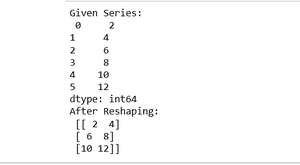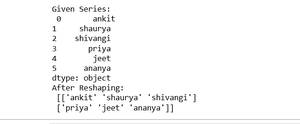# How to reshape Pandas Series?

• Last Updated : 01 Oct, 2020

In this article, we will see how to reshaping Pandas Series. So, for reshaping the Pandas Series we are using reshape() method of Pandas Series object.

Syntax: Pandas.Series.values.reshape((dimension))

Return: return an ndarray with the values shape if the specified shape matches exactly the current shape, then return self (for compat)

Let’s see some of the examples:

Example 1:

## Python3

 `# import pandas library ` `import` `pandas as pd ` ` `  `# make an array ` `array ``=` `[``2``, ``4``, ``6``, ``8``, ``10``, ``12``] ` ` `  `# create a series ` `series_obj ``=` `pd.Series(array) ` ` `  `# convert series object into array ` `arr ``=` `series_obj.values ` ` `  `# reshaping series  ` `reshaped_arr ``=` `arr.reshape((``3``, ``2``)) ` ` `  `# show  ` `reshaped_arr`

Output:Example 2:

## Python3

 `# import pandas library ` `import` `pandas as pd ` ` `  `# make an array ` `array ``=` `[``"ankit"``,``"shaurya"``, ` `         ``"shivangi"``, ``"priya"``, ` `         ``"jeet"``,``"ananya"``] ` ` `  `# create a series ` `series_obj ``=` `pd.Series(array) ` ` `  `print``(``"Given Series:\n"``, series_obj) ` ` `  `# convert series object into array ` `arr ``=` `series_obj.values ` ` `  `# reshaping series  ` `reshaped_arr ``=` `arr.reshape((``2``, ``3``)) ` ` `  `# show  ` `print``(``"After Reshaping: \n"``, reshaped_arr)`

Output:My Personal Notes arrow_drop_up
Recommended Articles
Page :